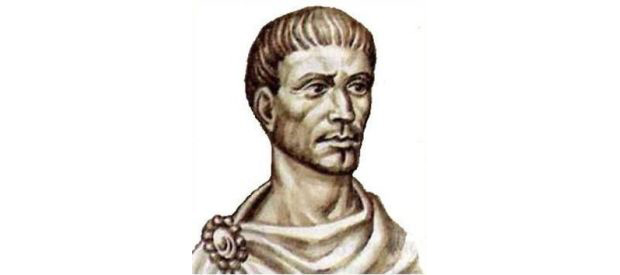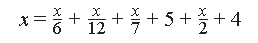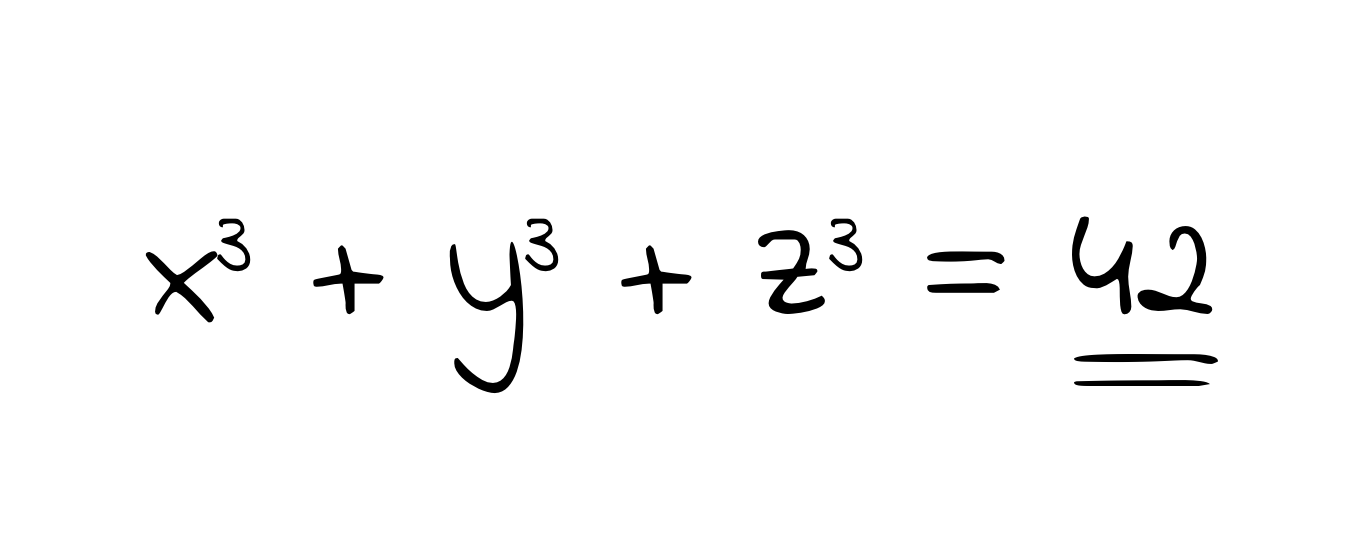Math & Beyond

# The Life of Diophantus of Alexandria

2.1k views

 1 Introduction 2 How Diophantus spent his early life 3 What is the Arithmetica? 4 What exactly is a Diophantine equation? 5 Diophantine Analysis: How to solve diophantine equations? 6 Death of Diophantus 7 Summary 8 FAQs

19 November 2020

## Introduction

Who is Diophantus?

What is Diophantus famous for?

Diophantus was an Alexandrian mathematician whose contributions to arithmetic are considered most essential to early mathematics. Mainly dealing with algebraic equations, his texts aim to validate and solve some of the most fundamental concepts of Number theory. He set forward several questions and proofs that led to the development of various important concepts including Fermat’s Last Theorem, approximate equalities, and decimals and fractions as coefficients in linear algebra.Source: Famous Mathematicians

## How Diophantus spent his early life

Born during AD 200 and 214 to 284 or 289 Diophantus lived in Egypt during the Roman Era. Little is known about the early life of the mathematician as he was forgotten in Western Europe during the so-called dark ages. Majority of his work, in the form of books, has also not been recovered. Most of the knowledge about the mathematician has been derived from the 5th-century Greek anthology of number games and puzzles created by Metrodorus.

## What is the Arithmetica?

Arithmetica is an ancient Greek text written by Diophantus in 3rd Century AD. It is a cluster of 130 Algebraic problems with numerical solutions of both determinate and indeterminate equations. It was originally composed as a set of 13 books, but only 6 books have survived.
The equations present in the text are known as Diophantine equations, and the methods of solving them are called Diophantine analysis.

A lot of mathematicians like Pierre de Fermat, Joseph-Louis Lagrange, and Leonhard Euler have worked on the contents of Arithmetica. It continues to have a profound impact on present-day mathematics.

### An example of a problem from the Arithmetica (sometimes called his epitaph)

'Here lies Diophantus,' the wonder behold.
Through art algebraic, the stone tells how old:
'God gave him his boyhood one-sixth of his life,
One twelfth more as youth while whiskers grew rife;
And then yet one-seventh ere marriage begun;
In five years there came a bouncing new son.
Alas, the dear child of master and sage
After attaining half the measure of his father's life chill fate took him. After consoling his fate by the science of numbers for four years, he ended his life.'According to the puzzle, Diophantus’ age can be expressed by the equation above. If solved, it yields 84 years. However, his actual age cannot be validated.

## What exactly is a Diophantine equation?

A diophantine equation is a polynomial, with two or more variables. A diophantine equation can have variables with any power. Diophantine curves have more unknowns than equations, i.e. most of the variables have multiple values. This results in the formation of curves on a graph.
Diophantus was one of the first people to introduce symbolism to Algebra. The study of Diophantine equations, and finding their solutions is called Diophantine analysis.Source: Medium

## Diophantine Analysis: How to solve diophantine equations?

Diophantine Analysis is the method of solving Diophantine Equations. A Diophantine Analysis is conducted with the following steps:

• Is it possible to get a solution?
• Is it possible to find solutions just by inspection?
• Are the number of solutions finite or infinite?
• Is it possible to obtain all the solutions?

## Death of Diophantus

Diophantus is said to have died between AD 285 and 299.

## Summary

This article talks about one of the greatest mathematicians to have graced the surface of the planet. His efforts and discoveries in algebra and number theory have been invaluable to advances in the field. Fermat’s last theorem, technique of adequality, maximas, minimas, and tangents of functions have all drawn inspiration from Diophantus’ work. The contributions he made have a profound impact on the world even today.

Get better at math with us, sign up for a free trial.

Cuemath, a student-friendly mathematics and coding platform, conducts regular Online Live Classes for academics and skill-development, and their Mental Math App, on both iOS and Android, is a one-stop solution for kids to develop multiple skills. Understand the Cuemath Fee structure and sign up for a free trial.

## What is Diophantus famous for?

He is famous for essential contributions to Number theory, including the Diophantine equations, the Fermat's last theorem, approximate equalities and the series of books authored by him titled Arithmetica.

## Where was Diophantus from?

He lived in Alexandria, Egypt. He is described as Greek or Egyptian.

## Who wrote Arithmetica?

Diophantus of Alexandria wrote a series of books titles Arithmetica.

## When did Diophantus die?

Somewhere around AD 284 or 298.

## What is Diophantine analysis?

Diophantine Analysis is the method of solving Diophantine Equations. A Diophantine Analysis is conducted with the following steps:

• Is it possible to get a solution?
• Is it possible to find solutions just by inspection?
• Are the number of solutions finite or infinite?
• Is it possible to obtain all the solutions?

## External References

Wikipedia.org - Diophantine Equations, Arithmetica

Related Articles
Award-winning math curriculum, FREE for a year